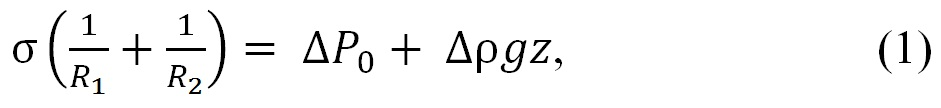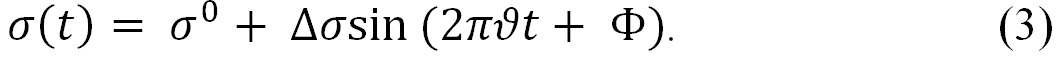Model PAT-1, SINTERFACE

# Profile Analysis Tensiometer (PAT)

Device for investigating rheological properties and interfacial tensions of surfactant solutions

### The physical principle

With the PAT-1 can be measured surface and interfacial tensions of liquids based on the analysis of the shape of pendant and growing drops.

The main principle of this method is to determine the surface tension of a liquid from the shape of a pendant or a growing drop. This shape is given by the Gauss-Laplace equation (1), which represents a relationship between the curvature of a liquid meniscus and the surface tension σwhere R1 and R2 are the main radii of curvature, ΔP0 is the pressure difference in a reference plane, Δρ is the density difference, g is the acceleration due to gravity, and z is the vertical height of the reference plane.

The surface tension σ can be determined by fitting the Gauss-Laplace equation to the coordinates of a drop or bubble, using σ as the fitting parameter.

To determine the rheological properties (dilational elasticity and viscosity)  of drops and/or bubbles one varies the area A (volume V ) harmonicallywhich, in turn, yields to a sinusoidal variation of the surface tension with an angular phase shift ΦThe oscillation of the drop/bubble is detected with a camera to calculate the variation of the surface tension as described above.

  Interfacial Transport Processes and Rheology, D. A. Edwards, H. Brenner, D. T. Wasan, Butterworth-Heinemann, Boston, 1991.Dr.

### Lukas Koch

#### Postdoctoral Research AssociateDr.